• 连通分量个数
千次阅读
2021-11-10 18:29:50

问题描述】
根据输入的图的邻接矩阵A，判断此图的连通分量的个数。

【输入形式】
第一行为图的结点个数n，之后的n行为邻接矩阵的内容，每行n个数表示。其中A[i][j]=1表示两个结点邻接，而A[i][j]=0表示两个结点无邻接关系。

【输出形式】
输出此图连通分量的个数。

【样例输入】
5
0 1 1 0 0
1 0 1 0 0
1 1 0 0 0
0 0 0 0 1
0 0 0 1 0

【样例输出】
2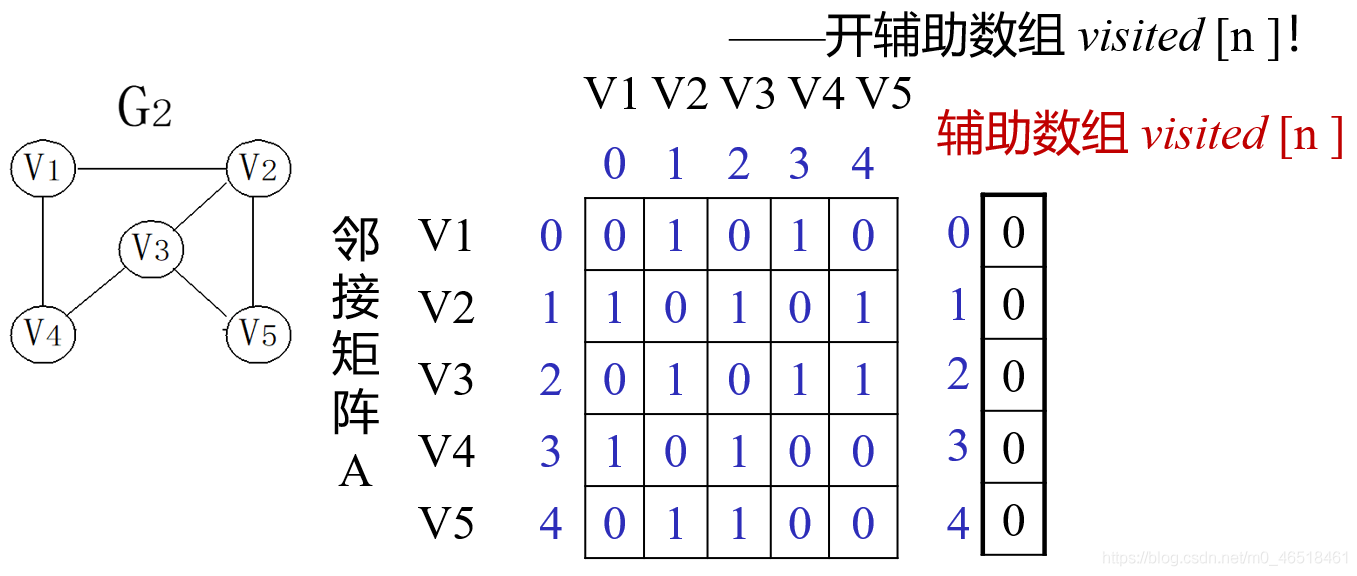所以咱们这么构思一下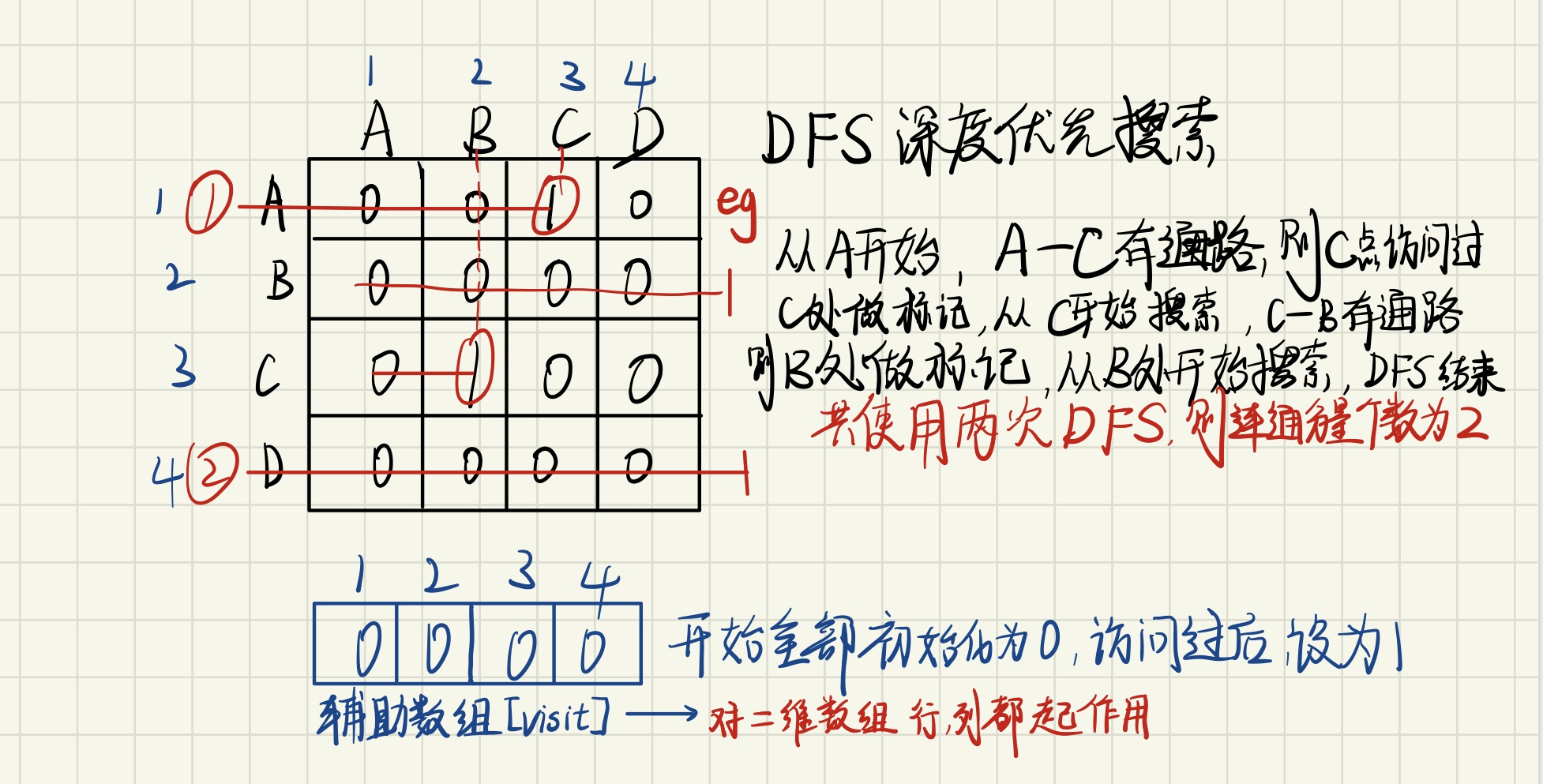#include<iostream>
using namespace std;
#define Maxsize 1024      //定义全局变量 数组visit[]
int visit[Maxsize];
typedef struct Graph
{
int a;        //建立邻接矩阵  Graph图类型
}Graph;
void Creat(int n,Graph *s)
{
int i, j;
for ( i = 0; i < n; i++)
{
for ( j = 0; j < n; j++)    //双循环 写入Graph s
{
cin >> s->a[i][j];
}
}
}
void Dfs(Graph *s,int visit[],int i,int n)  //此处传参i为行值
{
visit[i] = 1;      //设标记 已访问过
for (int j = 0; j < n; j++)         //内部循环（列） 寻找是否和行点有通路
{
if (!visit[j]&&s->a[i][j]==1)
//如果AB两点之间都没有被访问过 并且 A点和 A B C D点之间有连通
{
Dfs(s, visit, j, n);    //继续搜索
}
}
}           //此处Dfs搜索的意义在于：将访问过（可联通的）点做标记，通过调用外部一次性bfs的次数 判断连通分量的个数

void Makevisit(Graph *s,int visit[],int n)
{
int i, f = 0;
for (i = 0; i < n; i++)
visit[i] = 0;			//初始化辅助数列
for (i = 0; i < n; i++)     //外部循环是从第一个点（行上的点）（若访问过则不再访问）
{        // (确保所有的点都要访问，才能准确的判断连通分量个数)
if (!visit[i])           //判断该点是否遍历过 若未遍历过 则进入
{
f++;
Dfs(s, visit, i, n);
//调用搜索的次数 即为连通分量的个数
}
}
cout << f;
}

int main()
{
int n;
Graph *s=NULL;           //表s初始化为空
s = new Graph;       //给表s赋值空间
cin >> n;
Creat(n,s);              //创建表
Makevisit(s, visit, n);  //搜索
return 0;
}

更多相关内容
• ## 图的连通分量个数

千次阅读 2020-07-28 00:28:37
一、定义：         在无向图中，如果从顶点vi到顶点vj有路径，则称vi和vj连通。...上面有向图的连通分量个数为2 二、分析： 我们给图的每个结点设置一个访问标志，用visited

## 一、定义：

在无向图中，如果从顶点vi到顶点vj有路径，则称vi和vj连通。如果图中任意两个顶点之间都连通，则称该图为连通图，否则，将其中的较大连通子图称为连通分量。
在有向图中，如果对于每一对顶点vi和vj，从vi到vj和从vj到vi都有路径，则称该图为强连通图；否则，将其中的极大连通子图称为强连通分量。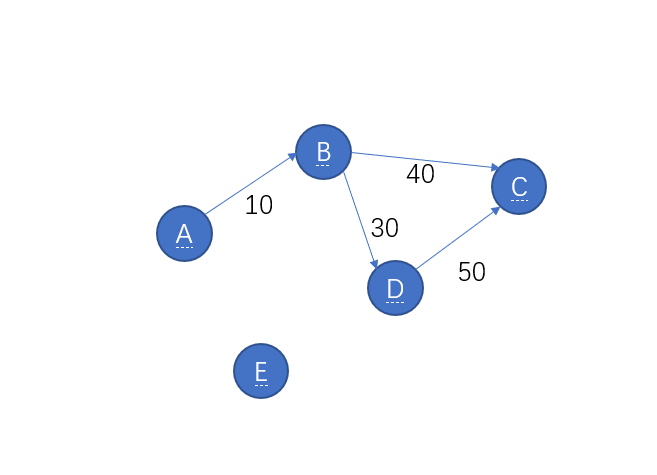上面有向图的连通分量个数为2

## 二、分析：

• 我们给图的每个结点设置一个访问标志，用visited[]数组来表示，0代表未访问，1代表已经访问
• 然后我们求从每个节点开始的深度优先遍历序列，每访问到一个结点，就将该结点对应的visited[]置1.
• 若当前结点已经被访问过(也就是visited[]数组中对应位置为1)，那这个结点就不用再深度优先遍历，
• 只有visited[]对应位置为0时才在当前结点进行深度优先遍历，深度优先遍历的次数就是该图的连通分量个数

## 三、核心算法：

typedef struct {
DataType list[MaxSize];
int size;
}SeqList;
typedef struct {
SeqList Vertices;   //存放顶点的顺序表
int edge[MaxVertices][MaxVertices];//存放边的邻接矩阵
int numOfEdges;            //边的条数

//这里假设图的顶点信息为字母类型
//连通图的深度优先遍历函数
void DepthFSearch(AdjMGraph G, int v, int visited[], void Visit(DataType item)) {
//连通图G以v为顶点的、访问操作为Visit()的深度优先遍历
//数组visited标记相应顶点是否已访问过(0表示未访问，1表示已访问)
int w;
Visit(G.Vertices.list[v]);//访问顶点v
visited[v] = 1;    //置已被访问标记
w = GetFirstVex(G,v);//取第一个邻接顶点
while (w != -1) {
if (!visited[w])
DepthFSearch(G, w, visited, Visit);  //递归
w = GetNextVex(G, v, w);     //取下一个邻接顶点
}
}
//非连通图的深度优先遍历函数（返回值为连通分量的个数）
int DepthFirstSearch(AdjMGraph G, void Visit(DataType item))
//非连通图G的访问操作为Visit()的深度优先遍历
{
int i;
int count = 0;
int *visited = (int *)malloc(sizeof(int)*G.Vertices.size);
for (i = 0; i < G.Vertices.size; i++) {
visited[i] = 0;
}
for (i = 0; i < G.Vertices.size; i++) {
if (!visited[i]){
count++;//统计连通分量的个数
DepthFSearch(G, i, visited, Visit);//以每个顶点为初始顶点进行调用
}
}
free(visited);
return count;
}


## 四、完整代码：

下面这个是有向图的代码，若要改为无向图，就将插入点和插入边的函数修改就行。

#pragma once
//图的邻接矩阵存储结构
#include "SeqList.h"
typedef struct {
SeqList Vertices;   //存放顶点的顺序表
int edge[MaxVertices][MaxVertices];//存放边的邻接矩阵
int numOfEdges;            //边的条数
//初始化
{
int i, j;
for(i=0;i<n;i++)
for (j = 0; j < n; j++) {
if (i == j) {
G->edge[i][j] = 0;
}
else {
G->edge[i][j] = MaxWeight;    //MaxWeight表示无穷大
}
}
G->numOfEdges = 0;      //边的条数置零
ListInitiate(&G->Vertices);   //顺序表初始化
}
//插入顶点
void InsertVertex(AdjMGraph *G, DataType vertex) {
//在途中插入顶点Vertex
ListInsert(&G->Vertices, G->Vertices.size, vertex);//在顺序表尾部插入
}
/*
插入边
在有向图中插入一条有向边。对于增加无向边的操作，可通过增加两条有向边完成。
*/
void InsertEdge(AdjMGraph *G, int v1, int v2, int weight) {
//在图中插入边<v1,v2>,边<v1,v2>的权为weight
if (v1 < 0 || v1 >= G->Vertices.size || v2 < 0 || v2 >= G->Vertices.size) {
printf("参数v1或v2越界出错!\n");
return;
}
G->edge[v1][v2] = weight;
G->numOfEdges++;
}
/*
删除边
在图中删除一条有向边。对于删除无向边的操作，可通过取消两条有向边完成
*/
void DeleteEdge(AdjMGraph *G, int v1, int v2) {
//在图中删除边<v1,v2>
if (v1 < 0 || v1 >= G->Vertices.size || v2 < 0 || v2 >= G->Vertices.size || v1 == v2) {
printf("参数v1或者v2越界出错!\n");
return;
}
if (G->edge[v1][v2] == MaxWeight || v1 == v2) {
printf("该边不存在!\n");
return;
}
G->edge[v1][v2] = MaxWeight;
G->numOfEdges--;
}
/*
取第一个邻接顶点
对于邻接矩阵来说，顶点v的第一个邻接顶点，就是邻接矩阵的顶点v行中
从第一个矩阵元素开始的非0且非无穷大的顶点
*/
//在图G中寻找序号为v的顶点的第一个邻接顶点
//如果这样的邻接顶点存在，则返回该邻接顶点的序号，否则返回-1
{
int col;
if (v < 0 || v >= G.Vertices.size) {
printf("参数v1越界出错");
return -1;
}
else {
for (col = 0; col < G.Vertices.size; col++) {
if (G.edge[v][col] > 0 && G.edge[v][col] < MaxWeight)
return col;
}
return -1;
}
}
/*
取下一个邻接顶点
对于邻接矩阵存储结构来说，顶点v1的邻接顶点v2的下一个邻接顶点，就是邻接矩阵的顶点
v行中从第v2+1个矩阵元素开始的非0且非无穷大的顶点
*/
int GetNextVex(AdjMGraph G, int v1, int v2) {
//在图中寻找v1的顶点的邻接顶点v2的下一个邻接顶点
//如果这样的邻接顶点存在，则返回该邻接顶点的序号，否则返回-1
//v1和v2都是相应顶点的序号
int col;
if (v1 < 0 || v1 >= G.Vertices.size || v2 < 0 || v2 >= G.Vertices.size) {
printf("参数v1或v2越界出错!\n");
return -1;
}
for (col = v2 + 1; col < G.Vertices.size; col++) {
if (G.edge[v1][col] > 0 && G.edge[v1][col] < MaxWeight)
return col;
}
return -1;
}



#pragma once
typedef struct {
int Row;   //行下标
int col;   //列下标
int weight;  //权值
}RowColWeight;   //边信息结构体定义
void CreateGraph(AdjMGraph *G, char V[], int n, RowColWeight E[], int e) {
//在图G中插入n个顶点信息V和e条边信息E
int i, k;
Initiate(G, n);    //顶点顺序表初始化
for (i = 0; i < n; i++)
InsertVertex(G, V[i]);//插入顶点
for (k = 0; k < e; k++)
InsertEdge(G, E[k].Row, E[k].col, E[k].weight);//插入边
}


#pragma once
#include"SeqList.h"
//这里假设图的顶点信息为字母类型
//连通图的深度优先遍历函数
void DepthFSearch(AdjMGraph G, int v, int visited[], void Visit(DataType item)) {
//连通图G以v为顶点的、访问操作为Visit()的深度优先遍历
//数组visited标记相应顶点是否已访问过(0表示未访问，1表示已访问)
int w;
Visit(G.Vertices.list[v]);//访问顶点v
visited[v] = 1;    //置已被访问标记
w = GetFirstVex(G,v);//取第一个邻接顶点
while (w != -1) {
if (!visited[w])
DepthFSearch(G, w, visited, Visit);  //递归
w = GetNextVex(G, v, w);     //取下一个邻接顶点
}
}
//非连通图的深度优先遍历函数（返回值为连通分量的个数）
int DepthFirstSearch(AdjMGraph G, void Visit(DataType item))
//非连通图G的访问操作为Visit()的深度优先遍历
{
int i;
int count = 0;
int *visited = (int *)malloc(sizeof(int)*G.Vertices.size);
for (i = 0; i < G.Vertices.size; i++) {
visited[i] = 0;
}
for (i = 0; i < G.Vertices.size; i++) {
if (!visited[i]){
count++;//统计连通分量的个数
DepthFSearch(G, i, visited, Visit);//以每个顶点为初始顶点进行调用
}
}
free(visited);
return count;
}


SeqList.h

#pragma once
typedef struct {
DataType list[MaxSize];
int size;
}SeqList;
//初始化
void ListInitiate(SeqList *L) {
L->size = 0;
}
//求当前数据元素个数
int ListLength(SeqList L) {
return L.size;
}
//插入数据元素
int ListInsert(SeqList *L, int i, DataType x) {
//在顺序表的第i个位置(0<=i<=size)个位置前插入数据元素值x
//插入成功返回1，插入失败返回0
int j;
if (L->size >= MaxSize) {
printf("顺序表已满无法插入!");
return 0;
}
else if (i<0 || i>L->size) {
printf("参数i不合法!\n");
return 0;
}
else {
//从后向前依次后移数据，为插入做准备
for (j = L->size; j > i; j--) {
L->list[j] = L->list[j - 1];
}
L->list[i] = x;
L->size++;
return 1;
}
}
//删除数据元素
int ListDelete(SeqList *L, int i, DataType *x) {
//删除顺序表L中第i(0<=i<=size-1)个位置处的数据元素并保存到x中
//删除成功返回1，删除失败返回0
int j;
if (L->size <= 0) {
printf("顺序表已空无法删除!\n");
return 0;
}
else if (i<0 || i>L->size - 1) {
printf("参数i不合法");
return 0;
}
else {
*x = L->list[i];//保存删除的元素到x中
//从前向后依次前移
for (j = i + 1; j <= L->size - 1; j++) {
L->list[j - 1] = L->list[j];
}
L->size--;
return 1;
}
}
//取数据元素
int ListGet(SeqList L, int i, DataType *x) {
//取顺序表的第i个数据元素存于x中，成功返回1，失败返回0
if (i<0 || i>L.size - 1) {
printf("参数i不合法!");
return 0;
}
else {
*x = L.list[i];
return 1;
}
}


test.cpp:

#pragma once
#include<stdio.h>
#include<stdlib.h>
typedef char DataType;
#define MaxSize 10
#define MaxVertices 10
#define MaxWeight 10000
#include "SeqList.h"
void Visit(DataType item) {//定义访问操作函数
printf("%c  ", item);
}
int main() {
DataType a[] = { 'A','B','C','D','E' };
RowColWeight rcw[] = { {0,1,10},{0,4,20},{1,3,30},{2,1,40},{3,2,50} };
int n = 5, e = 5;
int i, j;
CreateGraph(&g1, a, n, rcw, e);
//DeleteEdge(&g1, 0, 4);//删除边<0,4>
printf("顶点集合为:");
for (i = 0; i < g1.Vertices.size; i++) {
printf("%c  ", g1.Vertices.list[i]);
}
printf("\n");
printf("权值集合为:\n");
for (i = 0; i < g1.Vertices.size; i++) {
for (j = 0; j < g1.Vertices.size; j++) {
printf("%5d  ", g1.edge[i][j]);
}
printf("\n");
}
printf("***********************\n");
printf("深度优先遍历序列为:\n");
int count=DepthFirstSearch(g1,Visit);
printf("连通分量的个数为:%d\n", count);
system("pause");
}


## 五、运行结果：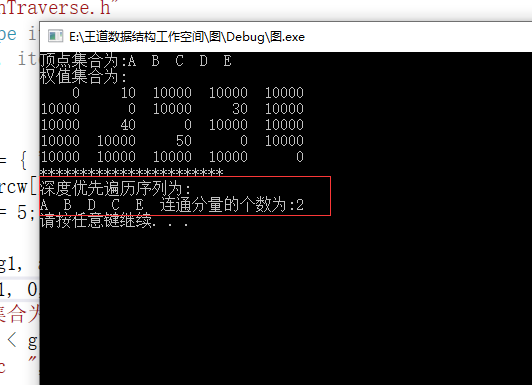展开全文数据结构 算法 链表 有向图 c语言
• 要求采用邻接矩阵作为无向图的存储结构，邻接表作为有向图的存储结构，完成无向图和有向图的建立，并对建立好的图进行深度和广度优先遍历。具体实现要求： 1. 通过键盘输入图的顶点...3. 统计两图的连通分量的个数。
• ## 求连通分量个数

千次阅读 2019-04-30 12:31:12
要求该图中连通分量个数，该图可以简化为两个节点之间的连线（整数对 p , q ） quick-find算法(O(N^2))，也是一般人最容易想到的算法 1. 用一个id数组来确定两个节点之间是否存在于相同的连通分量中 ， 保证同一...

如图所示：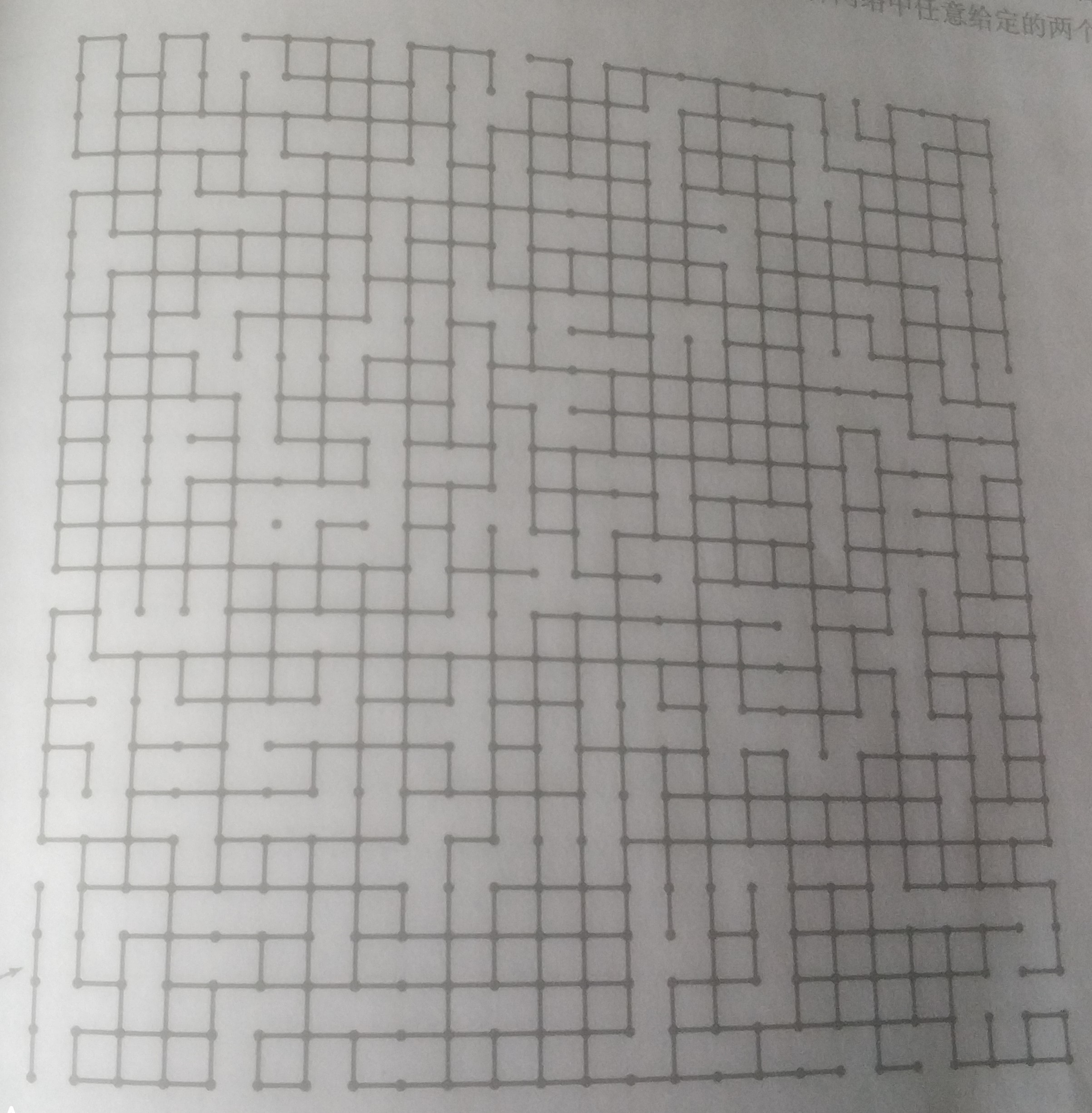要求该图中连通分量个数，该图可以简化为两个节点之间的连线（整数对 p , q ）

# quick-find算法(O(N^2))，也是一般人最容易想到的算法

1. 用一个id数组来确定两个节点之间是否存在于相同的连通分量中 ， 保证同一个连通分量的所有节点的在id数组中的值全部相同（id数组记录的是p点所在连通分量的标号）

2. 先用 find函数 判断p , q是否在同一个相同分量，如果是，则不做任何操作，如果不在，将p , q所在的连通分量用  union 函数连接起来

int count = id.length;        //记录连通分量个数

public int find(int p)
{
return id[p];
}

public void union (int p,int q)  //将p，q归并到相同的分量
{
int pID = find(p);
int qID = find(q);

if(pID == qID)   //如果已经是在同一个分量，不做任何操作
return;
for(int i = 0; i < id.length; i++)
if(id[i] == pID)
id[i] = qID;

count--;    //分量个数减1
}

但是上述算法必须每次扫描原来的id数组，再改变 p , q的对应id值，这样太过于复杂，所以有了下面算法

# quick-union算法（O(N^2)）

为了提高union函数的速度，可以在实现find方法时，从给定的节点开始，由它的链接得到另一个节点(id数组不再是记录p点所在的连通分量标号，而是记录p点的上一个节点，类似于链表)，一直下去，直到找到根节点，将两个根节点直接连接在一起即可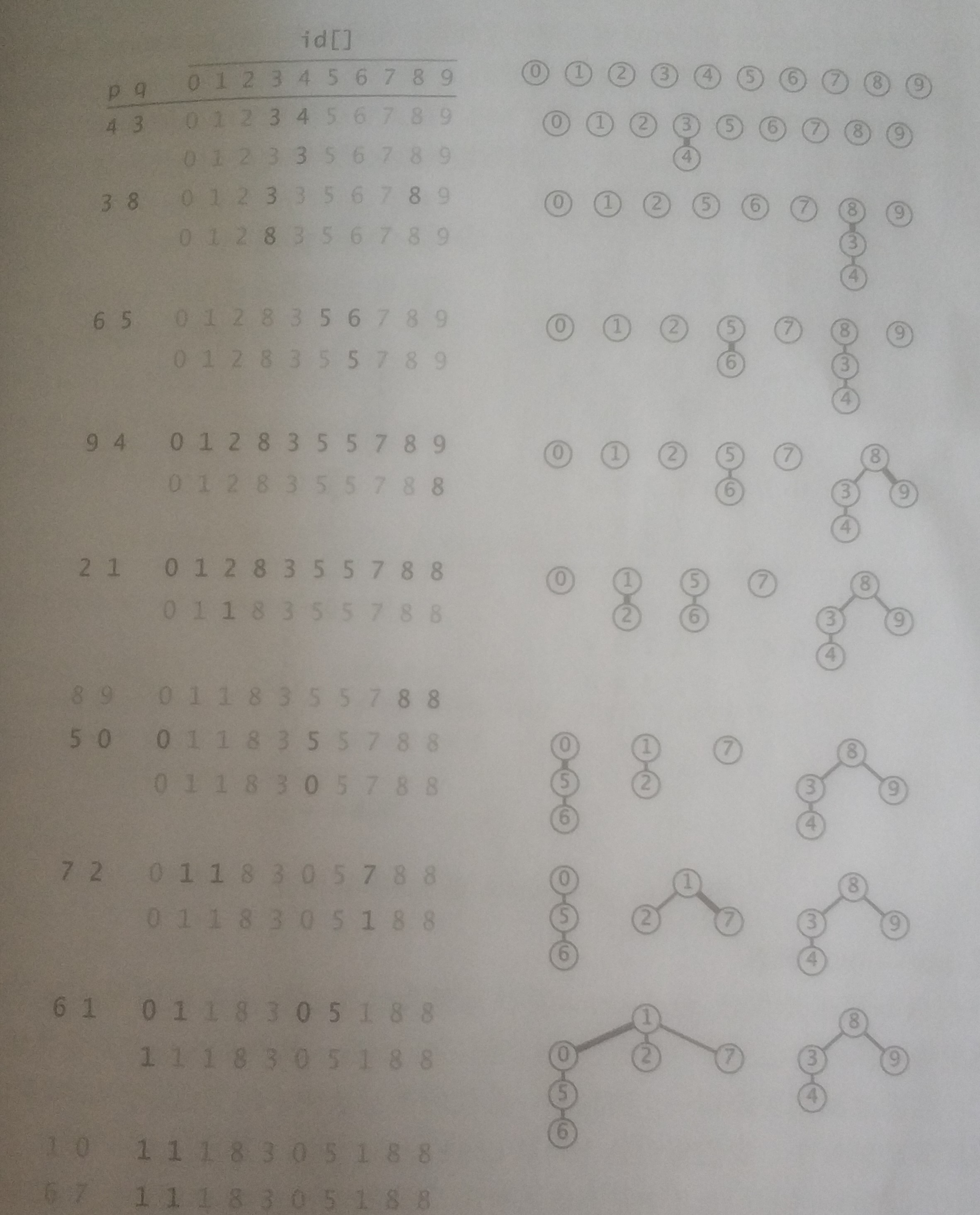int count = id.length;             //  记录连通分量个数

for(int i = 0; i < id.length; i++)      //  初始化所有节点的根节点为自身
{
id[i] = i;
}

int find(int p)              //  类似链表，直到找到根节点为止
{
while(p != id[p])
p = id[p];
return p;
}

void union(int p, int q)
{
int pRoot = find(p);
int qRoot = find(q);
if(pRoot == qRoot)
return ;
id[pRoot] = qRoot;       //  将两个根节点连接
count--;                 //  连通分量个数减1
}

quick-union算法看上去比quick-find算法快，因为它不需要对每个输入都遍历id数组，在最好的情况下，find()只需要访问一次数组即可找到根节点，但是最坏情况下需要访问2*N + 1次，所以需要的复杂度也是O(N^2) , 但是比quick-find要快

# union-find(加权quick-union算法  , O(n * logn) , 目前较好的算法)

在quick-union算法中，较坏情况下是在一条较长的链中寻找根节点，所以在该算法中，手动将较小的链加到较大的链中，而不是像上面算法一样无脑的连接起来，以达到平衡每个树的节点个数尽可能相等

public class WeightedQuickUnionUF
{
private int [] id;          //  记录该节点的根节点信息
private int [] sz;          //  记录各个节点所对应的分量大小，即每条链的节点数
private int count;

public WeightedQuickUnionUF(int N)
{
count = N;
id = new int [N];
for(int i = 0; i < N; i++)       //  初始化节点的根节点为自身
id[i] = i;
for(int i = 0; i < N; i++)       //  初始化每个链的节点个数为1
sz[i] = 1;
}

public int find(int p)
{
while(p != id[p])
p = id[p];
return p;
}

public void union(int p,int q)
{
int i = find(p);
int j = find(q);
if(i == j)
return;
if(sz[i] < sz[j])
{
id[i] = j;            //  将较小的链连接到较大的链上(更新小链的根节点的id值为
//  较大链的根节点)
sz[j] += sz[i];       //  更新链的长度，即节点个数
}
else
{
id[j] = i;
sz[i] += sz[j];
}

count--;                  //  连通分量个数减1

}
}

# 带路径压缩的加权quick-union算法（O(n*logn) , 目前最优的算法）

基于上述加权quick-union算法 ， 在理想情况下，我们希望每个节点都直接连接到它的根节点，但是又不像quick-find算法那样通过修改大量连接达到该目的，所以只需在find()方法中添加一个循环，让在路径上遇到的所有节点的id值变成所在链的根节点，这样得到的一棵树是一颗几乎完全扁平化的树

public int find(int p)
{
int root = id[p];
while(root != id[root])
root = id[root];

while(p != root)
{
int q = id[p];
id[p] = root;
p = q;
}

return root;
}

展开全文# 一、利用dfs求图连通分量

• 初始化连通分量个数为ccount=0；
• 从图中任一顶点开始进行dfs，通过该顶点遍历到的所有顶点属于同一连通分量，这些遍历到的顶点做好标记，表示已经被访问。ccount+=1；
• 从未访问过的顶点中取出一个顶点重复第二步，遍历完后ccount+=1；
• 重复上述过程，直到所有顶点均被标记；
• 输出ccount，ccount即为图连通分量个数。
#include<bits/stdc++.h>
using namespace std;

class graph {
public:
vector<vector<int> > g;  // 图的领接表
graph(int V, bool directed=false){
this->V=V;  // 需传入图的顶点数
this->directed = directed;  // 是否为有向图
// g初始化为V个空的vector, 表示每一个g[i]都为空, 即没有任和边
g = vector<vector<int> >(V, vector<int>());
}
~graph(){};
void addEdge(int v, int w);  // 顶点v与w有一条边
int getV() { return V; }  // 取出顶点数
int getE() { return E; }  // 取出边数

private:
int V = 0;  // 顶点数
int E = 0;  // 边数
bool directed;  // 是否为有向图

};

void graph::addEdge(int v, int w) {
assert(v>=0 && v<V);
assert(w>=0 && w<V);
g[v].push_back(w);
if(!directed) g[w].push_back(v);  // 若不是有向图则w与v也有一条边
E++;
}


class Component {
private:
graph &G;  // 图的引用
bool *visited;  // 记录节点是否被访问
int ccount;  // 连通分量个数
int *id;  // 给每个顶点所属的连通分量标号

void dfs(int v);  // 从顶点v开始进行dfs
public:
Component(graph &graph): G(graph)
{
visited = new bool[G.getV()];
id = new int[G.getV()];
ccount = 0;
for (int i = 0; i < G.getV(); ++i) {
visited[i] = false;
id[i] = -1;
}

for (int i = 0; i < G.getV(); ++i) {
if(!visited[i])
{
dfs(i);
ccount++;
}
}
}

~Component(){
delete[] visited;
delete[] id;
}

// 返回连通分量个数
int count()
{
return ccount;
}

// 查询v和w是否连通
bool isConnected(int v, int w)
{
return id[v]==id[w];
}

// 以文字形式显示图的连通分量具体情况
void verbose()
{
for (int i = 1; i <= ccount; ++i) {
cout << "Component " << i << " Vertex:";
for (int j = 0; j < G.getV(); ++j) {
if(id[j] == i-1) cout << " " << j;
}
cout << endl;
}
}
};

void Component::dfs(int v) {
visited[v] = true;
id[v] = ccount;
// 遍历图G的顶点v的领接表
for (int i = 0; i < G.g[v].size(); ++i) {
if(!visited[G.g[v][i]])
dfs(G.g[v][i]);
}
}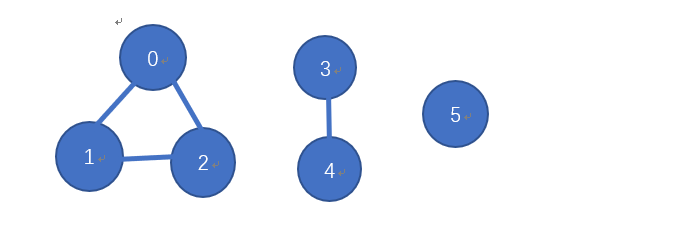int main()
{
graph G(6, false); // 建立顶点个数为6的图

Component component(G);
cout << "连通分量个数：" << component.count() << endl;  // 打印连通分量个数
cout << "1，2点是否连通：" << component.isConnected(1, 2) << endl;  // 判断两点是否连通
cout << "1，3点是否连通：" <<component.isConnected(1, 3) << endl;
component.verbose();  // 显示具体信息
return 0;
}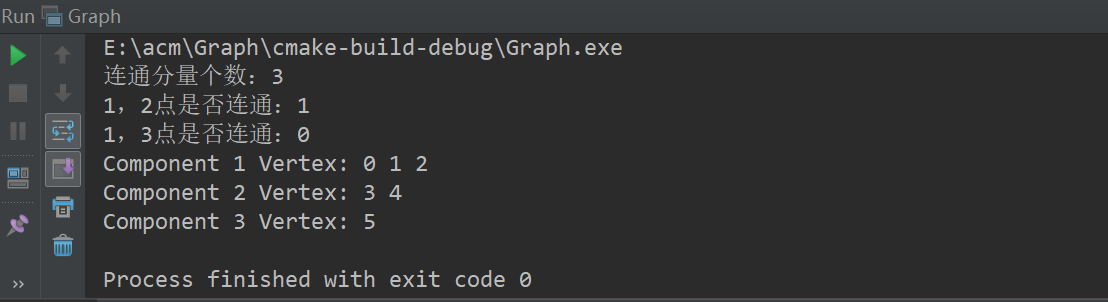# 二、并查集求连通分量个数

//
// Created by 程勇 on 2019/3/7.
//

/*
* 并查集可用来求图连通分类和最小生成树
*/
#include<bits/stdc++.h>
using namespace std;

const int maxn = 1e+6+10;
int parent[maxn];

int Find(int p)
{
while(p != parent[p])
{
p = parent[p];
}
return p;
}

void Union(int p, int q)
{
if(Find(p) != Find(q))
parent[p] = q;
}

int main()
{
int n, m;  // 顶点数和边数
cin >> n >> m;
for (int i = 1; i <= n; ++i) {
parent[i] = i;  // 初始化每个顶点指向自己
}
for (int j = 0; j < m; ++j) {
int v, w;
cin >> v >> w;  // 依次读入边
v = Find(v);
w = Find(w);
Union(v, w);
}

int res = 0;
for (int i = 1; i <= n; ++i) {
if(parent[i] == i) res++;
}

cout << "连通分量数：" << res-1 << endl;
system("pause");
return 0;
}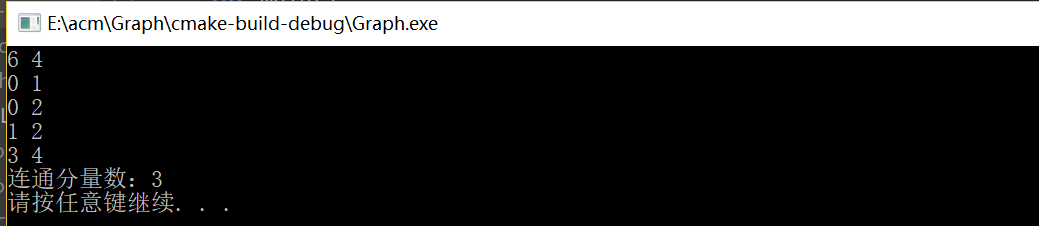展开全文• ## 强连通分量个数的求法(图解）

万次阅读 多人点赞 2020-09-27 01:30:11
连通分量个数的求法（图解） 背景 最近刷软考题的时候，碰到2013年上半年软件设计师的第31题，求程序图的环路复杂度。答案解析中有这么一段话： 根据图论，在一个强连通的有向图G中，环的个数V(G)由以下公式给出...
• 数据结构算法—邻接表存储的无向图求连通分量个数 邻接表存储结构 typedef struct ArcNode{ int adjvex;//边指向的顶点 struct ArcNode *nextarc; // 下一条边的指针 }ArcNode; typedef struct VNode{ int data;...数据结构 算法
• 【LeetCode】﹝并查集ி﹞连通分量个数(套用模板一直爽) 文章目录【LeetCode】﹝并查集ி﹞连通分量个数(套用模板一直爽)模板（使用路径压缩的加权quick-union算法）连通网络的操作次数★★省份数量★★岛屿数量★★...leetcode 并查集 数据结构
• 有向图的连通分量的求解思路 kosaraju算法 逛了很多博客，感觉都很难懂，终于找到一篇能看懂的，摘要记录一下 原博客https://www.cnblogs.com/nullzx/p/6437926.html 关于连通分量是什么自行百度，这里主要...kosaraju算法
• 求图的连通分量个数在算法题中时有考到，是图算法中基础而重要的问题。对于这个问题，常用的解法有搜索算法（DFS、BFS等），及并查集算法。图的搜索算法在我的其他博文中已经介绍过，这里用一个例题介绍一下并查集求...算法
• 数据结构实验：连通分量个数Problem Description 在无向图中，如果从顶点vi到顶点vj有路径，则称vi和vj连通。如果图中任意两个顶点之间都连通，则称该图为连通图， 否则，称该图为非连通图，则其中的极大连通...数据结构
• 题目描述 从键盘接收图的顶点集，关系集，创建无向图。 第一行依次输入图的顶点个数n，关系个数k，以空格隔开...计算连通分量个数并输出。 输出一个整数，表示连通分量个数。 样例输入 6 7 ABCDEF AB AE BC CD DA ...
• 问题 B: DS_7.2 求解连通分量个数(by Yan) 问题 B: DS_7.2 求解连通分量个数(by Yan)题目描述从键盘接收图的顶点集，关系集，创建无向图。 第一行依次输入图的顶点个数n，关系个数k，以空格隔开。顶点个数&lt;=...
• 1：DFS #define N 10000 //图中点的个数 int graph[N][N];// 0 表示没有边 1 表示有边 bool visited[N]={false};...void DFS(int root){ ...将能相互连通的点的集合赋予一共同的父亲，统计父亲的个数就行了。
• 给出一图的邻接矩阵，对图进行深度优先搜索，从顶点0开始   class Graph { private: int flag[N];//状态组 int Vexnum;//图的顶点数量 int Matrix[N][N]; //邻接矩阵 void DFS(...
• 数据结构实验：连通分量个数 Time Limit: 1000 ms Memory Limit: 65536 KiB Submit Statistic Problem Description  在无向图中，如果从顶点vi到顶点vj有路径，则称vi和vj连通。如果图中任意两个顶点之间都...
• ## 连通分量个数

千次阅读 2014-08-26 20:49:11
否则，称该图为非连通图，则其中的极大连通子图称为连通分量，这里所谓的极大是指子图中包含的顶点个数极大。 例如：一个无向图有5个顶点，1-3-5是连通的，2是连通的，4是连通的，则这个无向图有3个连通分量。 求...
• 问题 B: DS_7.2 求解连通分量个数(by Yan) 题目描述 从键盘接收图的顶点集，关系集，创建无向图。 第一行依次输入图的顶点个数n，关系个数k，以空格隔开。顶点个数<=20 第二行依次输入顶点值，类型为字符。 接...
• /// 这里是用 vector 存图 .../// vis[] 用来标记这点是否被访问过 void dfs(int p,int &sz){ for(int i = 0;i < v[p].size();i ++){ int son = v[p][i]; if(!vis[son]){ vis[son] = 1; ...
• 否则，称该图为非连通图，则其中的极大连通子图称为连通分量，这里所谓的极大是指子图中包含的顶点个数极大。 例如：一个无向图有5个顶点，1-3-5是连通的，2是连通的，4是连通的，则这个无向图有3个连通分量。   ...
• 数据结构实验：连通分量个数 Time Limit: 1000MS Memory limit: 65536K 题目描述  在无向图中，如果从顶点vi到顶点vj有路径，则称vi和vj连通。如果图中任意两个顶点之间都连通，则称该图为连通图， 否则，...数据结构 c++ 并查集
• ## 连通分量个数（并查集的应用）

千次阅读 多人点赞 2016-08-17 15:23:25
并查集是我暑假从高手那里学到的一招，觉得真是太精妙的设计了。以前我无法解决的一类问题竟然可以用如此简单高效的方法搞定。不分享出来真是对不起...最后要解决的是整幅图的连通性问题。比如随意给你两点，让你判
• 数据结构实验：连通分量个数 Time Limit: 1000MS Memory Limit: 65536KB Submit Statistic Discuss Problem Description  在无向图中，如果从顶点vi到顶点vj有路径，则称vi和vj连通。如果图中任意两......